• 零、注意要素 1、在迷宫周围需要设置围墙（一圈的1） 2、每个点的走动，需要记录方向，（x，y，方向） 3、如果某点遇到围堵，回退，那么需要标记这个点是不通的。会退后的下一点再往该方向...
零、注意要素 1、在迷宫周围需要设置围墙（一圈的1） 2、每个点的走动，需要记录方向，（x，y，方向） 3、如果某点遇到围堵，回退，那么需要标记这个点是不通的。会退后的下一点再往该方向查找时，这一点是不通的，那么那一点需要判断其他方向，如果其他方向也不同则回溯，并且标记那一点也为不通的。 4、stack的topPointer问题，topPointer是指向最上面的数据的上一个空间，
void PUSH(stackNode node,mazeStack *stack)
{
stack->nodeList[stack->top++]=node;
}
所以说当判断当前是否为空时，则如果top 的值为初始化的值，则为空。例如初始化为1，那么当top为1时，则栈为空，因为如果有一个元素的话，执行了stack->nodeList[stack->top++]=node;后top的值为2。 一般top初始化为0，所以判断空时，判断小于等于0；
int Empty(mazeStack *stack)
{
if(stack->top<=0)
{
return TRUE;
}
return FALSE;
}
这样可以理解如果退栈是，需要先将top-1，然后读取当前栈的数据，这样就少了一个数据，同时top仍然指向第一个元素的上一个位置。
int  POP(stackNode *node, mazeStack *stack)
{
if(stack->top<=0)
return FALSE;
*node=stack->nodeList[--stack->top];
return TRUE;
}
5、在这个代码中 Mask 和 Stack是不冲突的，他们之间是没有直接关联，即使Mask节点置为1，stack仍然能够退栈（这是当时迷糊很久的事，应该看到他们的独立性）
一、找路的思想    伪代码如下
将入口点压栈； while(堆栈不为空）
{
POP得到栈顶的元素1；
for(从 向右；一直到向上  ；逆时针更改方向）
{
得到新的坐标
if（不是墙壁 而且 没有来过）
{
将之前的位置记录(元素1）压栈;
踩过去，将当前的位置记录 压栈。
if（到达出口）
返回
退出 方向 for循环。
}
}
}

二、源代码
#include "stdafx.h"
#include <stdio.h>
#include <stdlib.h>
/*需要注意的是：当为0 的不同情况，需要有另外的标志表示它是不同的，要不然会老早重复的错误路径*/
#define MAZE_SIZE 5
#define START_X 1
#define START_Y 1
#define END_X MAZE_SIZE
#define END_Y MAZE_SIZE
#define DONE 0
#define NOTDONE -1
#define TRUE 0
#define FALSE -1
#define FRESH 0
#define OLD 1
#define DIRECTION_MAX_COUNT 4
typedef enum{Right,Down,Left,Up}Direction;
typedef enum{YES,NO}MARK_TAG;
int Maze[MAZE_SIZE+2][MAZE_SIZE+2];
int Mask[MAZE_SIZE+2][MAZE_SIZE+2];
typedef struct{
Direction dir;
int dx;
int dy;
}moveType;
moveType Move;
typedef struct {
int x;
int y;
}Pos;
typedef struct{
Pos pos;
Direction direction;
int order;
}stackNode;
typedef struct{
stackNode *nodeList;
int top;
int bottom;
}mazeStack;
void initStack(mazeStack *stack)
{
stack->nodeList=(stackNode * )malloc((MAZE_SIZE*MAZE_SIZE+1)*sizeof(stackNode));//top=0 is empty and is the empty test pos
stack->top=0;
stack->bottom=0;
}
void PUSH(stackNode node,mazeStack *stack)
{
stack->nodeList[stack->top++]=node;
}
int  POP(stackNode *node, mazeStack *stack)
{
if(stack->top<=0)
return FALSE;
*node=stack->nodeList[--stack->top];
return TRUE;
}
int Empty(mazeStack *stack)
{
if(stack->top<=0)
{
return TRUE;
}
return FALSE;
}

Pos getNextPos()
{
Pos nextPos;

return nextPos;
}
int findThePath(mazeStack * stack)
{
Pos curPos;
curPos.x=START_X;
curPos.y=START_Y;
int order=1;
/*pseudo code belowe*/
Pos nextPos;
stackNode node;
node.pos=curPos;
node.direction=Right;
node.order=order++;
PUSH(node,stack);
Mask[node.pos.x][node.pos.y]=OLD;
int dx,dy,dr,g,h;
Direction dir;
int i;
/*pseudo code  */
/* 将入口点压栈；
while(堆栈不为空）
{
POP得到栈顶的元素1；
for(从 向右；一直到向上  ；逆时针更改方向）
{
得到新的坐标
if（不是墙壁 而且 没有来过）
{
将之前的位置记录(元素1）压栈;
踩过去，将当前的位置记录 压栈。
if（到达出口）
返回

退出 方向 for循环。
}
}
}
/* */
while(Empty(stack)==FALSE)
{
if(POP(&node,stack)!=FALSE)
{
node.order=--order;
for(i=0;i<DIRECTION_MAX_COUNT;i++)
{
g=node.pos.x+Move[i].dx;
h=node.pos.y+Move[i].dy;
dir=Move[i].dir;

if(Maze[g][h]==0 && Mask[g][h]==FRESH)
{
node.direction=dir;
PUSH(node,stack);

Mask[g][h]=OLD;
node.pos.x=g;
node.pos.y=h;
node.direction=Right;
node.order=order++;
PUSH(node,stack);
if(g==END_X &&h==END_Y)
return TRUE;
break;
}
}

}
}

return FALSE;
}
void initMaze()
{
/*creat the outside wall*/
for(int i=0;i<MAZE_SIZE+2;i++)
{  Maze[i]=1;
Maze[MAZE_SIZE+1][i]=1;
}
for(int j=1;j<MAZE_SIZE+1;j++)
{
Maze[j]=1;
Maze[j][MAZE_SIZE+1]=1;
}
/**/
for(int i=1;i<MAZE_SIZE+1;i++)
for(int j=1;j<MAZE_SIZE+1;j++)
{
scanf("%d",&Maze[i][j]);
}
}
void initMask()
{
for(int i=0;i<MAZE_SIZE+2;i++)
for(int j=0;j<MAZE_SIZE+2;j++)
Mask[i][j]=FRESH;
}
void initMove()
{
Move.dir=Right;
Move.dx=0;
Move.dy=1;

Move.dir=Down;
Move.dx=1;
Move.dy=0;

Move.dir=Left;
Move.dx=0;
Move.dy=-1;

Move.dir=Up;
Move.dx=-1;
Move.dy=0;
}
int main()
{   mazeStack stack;

initMaze();
initMask();
initMove();
initStack(&stack);
if(findThePath(&stack)==TRUE)
{
for(int i=stack.bottom;i<stack.top;i++)
printf("(%d,%d)/n",stack.nodeList[i].pos.x-1,stack.nodeList[i].pos.y-1);
}
else
{
printf("No FInd/n");
}
scanf("%d",&Maze);
return 0;
}

三、运行效果
1、有通路情况2、无路情况四、可改进部分 1、迷宫的size，以及将方形的迷宫可以改为矩形的迷宫（不一定是正方形）     这些可以更改MAZE_SIZE宏、END_X、END_Y来达到，并添加长度、宽度 2、可以改进方向，由4个方向，改为8个方向。     typedef enum{Right,Down,Left,Up,NorthEast,SouthEast ,SouthWest,NorthWest}Direction;    #define DIRECTION_MAX_COUNT 8   修改void initMove()以配合8个方向。

展开全文语言 c struct
• 首先实现一个以链表做存储结构的栈类型，然后编写一个求解迷宫非递归程序。求得的通路以三元组(i，j，d)的形式输出，其中：(i，j)指示迷宫中的一个坐标，d表示走到下一坐标的方向。如：对于下列数据的迷宫，输出的...
• 通过利用c语言的设计一个关于迷宫问题，本设计程序实现一个以链表作存储结构的栈类型，再用非递归法走出迷宫的路径。
• 非递归求解迷宫问题问题解决的实现数据测试正常数据测试异常数据测试有疑问看这里 更新一下以往的课程设计，希望能给相同课程设计的同学提供一个不一样的思路。 问题解决的实现 问题描述：  迷宫问题一般使用地方...
非递归求解迷宫问题问题解决的实现运行环境说明：数据测试正常数据测试异常数据测试有疑问看这里源码及课程设计报告
更新一下以往的课程设计，希望能给相同课程设计的同学提供一个不一样的思路。
问题解决的实现

问题描述：
迷宫问题一般使用地方方式求解，当然也可以使用非递归回溯求解。
本次课程设计的目的就是通过非递归的方式求解迷宫问题，并完成过程的详细解释。

设计步骤：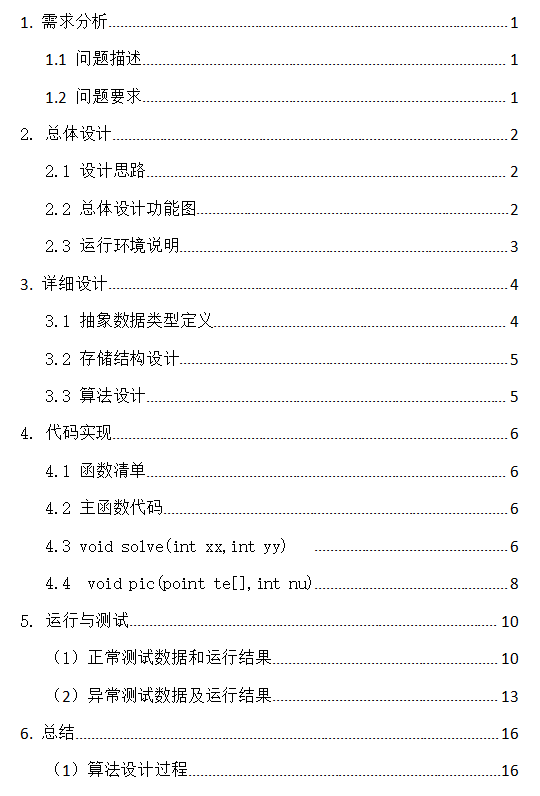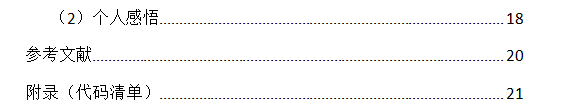算法设计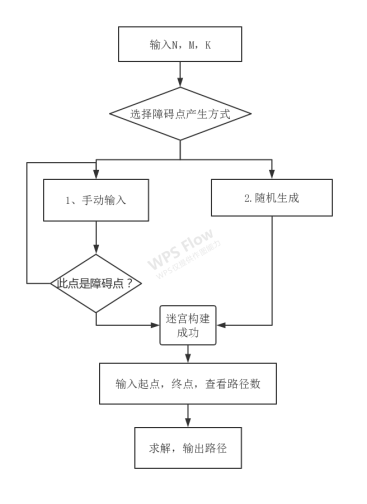主要变量

int: x={0,-1,0,1},y={-1,0,1,0};     //记录方向左上右下
Maxsize 101  ;                    //定义最大值
a[Maxsize][Maxsize];             //定义迷宫的模型
rows,cols,n,summ=0,minn=10001,want=-1;
//定义行列,障碍点个数,路径个数,最短路径的初始长度和显示的路径
every;                //是否每次按键显示整条路径
top=-1;              //初始化栈顶
bool :had[Maxsize][Maxsize];              //地图各点是否走过
Struct：
struct
{
int a;                        //坐标点 是否是可行性路径中的点
int d;                       //方向
}ans[Maxsize][Maxsize];
typedef struct
{
int x;
int y;
int k;
}point;                            //定义点的结构体
point path,sp;   //路径和最短路径


函数清单

void input();                    //输入迷宫的行列和障碍点数
void solve(int xx,int yy);          //非递归求解函数（xx,yy）为起点
void pic(point te[],int nu);        //打印出路径的图形,te为要打印的点的数组，nu为te中的元素个数
void check();                //检查有无通路及最短路径的输出
void bande();                     //输入起点，终点并打印迷宫
void shortpath();                  //求出最短路径


主函数代码

int main()
{
input();         //输入
solve(bx,by);    //求解
check();        //检查
return 0;
}


求解函数

void solve(int xx,int yy)   //非递归求解函数
{
int k;                     //定义方向变量
top++;
path[top].x=xx;
path[top].y=yy;
path[top].k=-1;               //起点入栈
had[xx][yy]=true;             //改变起点可走的状态
while(top>-1)    //栈不空循环
{
int nowx,nowy,i,j;
nowx=path[top].x;
nowy=path[top].y;
k=path[top].k;
if(nowx==ex&&nowy==ey)         //检查目前栈顶元素是否为终点
{
summ++;                     //可行性路径的总个数+1
if(top<minn) shortpath();     //由于每增加一个元素，top+1，故top的值可以表示各个路径的总长度，用以求出最短的一条路径

if(summ==1)  printf("  可供参考的迷宫可达路径为下:\n\n");
for(int t=0;t<top-1;t++)
printf("(%d,%d)->",path[t].x,path[t].y);
printf("(%d,%d)\n",path[top].x,path[top].y);  //路径的坐标输出
pic(path,top);    //调用函数pic，对路径进行图形输出
if(summ==want)   return ; //如果选择了想要查看的路径条数，解够了就终止
had[path[top].x][path[top].y]=false; //更改栈顶元素的可走状态
top--;
nowx=path[top].x;      //回溯发起另一个方向的求解
nowy=path[top].y;
k=path[top].k;
}
int find=0;
while(k<4&&!find){            //如果K在四个方向之内并且一直没找到下一步的路径就循环
k++;
if(k==4) break;
i=nowx+x[k];       //下一步的 坐标
j=nowy+y[k];
if(a[i][j]&&!had[i][j])           //如果（i，j）可以走并且没有走过
{
find=1;
path[top].k=k;           //改变栈顶元素的方向（用于打印方向）
top++;
path[top].x=i;
path[top].y=j;
path[top].k=-1;
had[i][j]=true;              //（i，j）入栈，并改变可走状态
}
}//while
if(!find){                            //如果一直没有找到下一步的位置
had[path[top].x][path[top].y]=false;
top--;                      //改变当前点的可走状态，并删除当前点
}
}
}


可视化打印

 void pic(point te[],int nu)
{
for(int i=0;i<nu;i++)
{    ans[te[i].x][te[i].y].a=1;
ans[te[i].x][te[i].y].d=te[i].k;            //将路径中各点在ans中赋值
}
ans[ex][ey].a=1;                //由于终点不在te中，需独立赋值
for(int i=0;i<=rows+1;i++)
{       printf("                               ");
for(int j=0;j<=cols+1;j++)
if(a[i][j]&&ans[i][j].a){
if(i==bx&&j==by||i==ex&&j==ey) {
printf("●"); continue;      //起点和终点用●打印
}
switch(ans[i][j].d){
case 0: printf("←");break;
case 1: printf("↑");break;
case 2: printf("→");break;
case 3: printf("↓");break;
}                         //根据ans[i][j].d的值来确定方向
}
else if(!a[i][j]) printf("▇") ;   //障碍点用▇打印
else printf("  ");
printf("\n");
}
for(int i=0;i<nu;i++)
{
ans[te[i].x][te[i].y].a=0;
ans[te[i].x][te[i].y].d=-1;
}                                       //一条路径打印完后进行初始化
if(every) system("pause") ;   //如果选择按键显示每条路径，暂停
cout<<endl;
}

运行环境说明：
由于调用函数default_random_engine ，所以我们的编译环境选项应调整为 ISO  C++ 11 。
Dev-c++中调整如下：打开工具（tools），选择编译选项（Compiler Options）,选择代码生成/优化（setting），点击代码生成（Code  Generation）,将语言标准（Language standard）改为ISO   C++ 11。
数据测试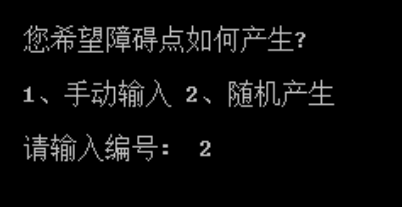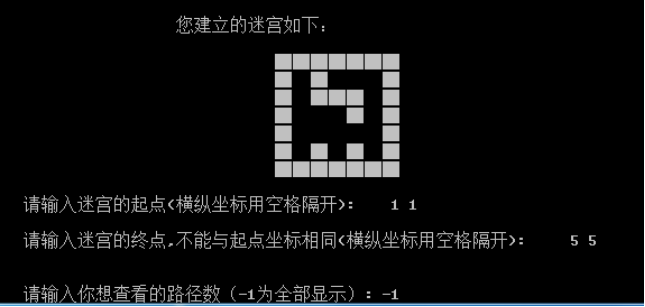正常数据测试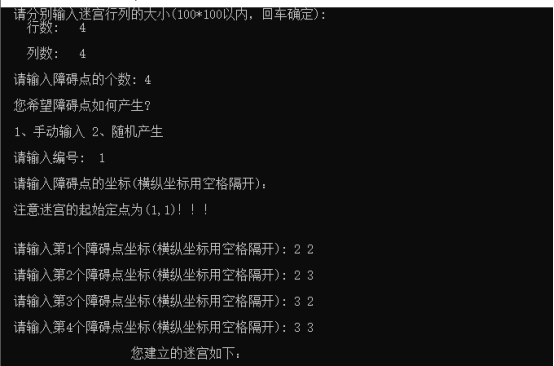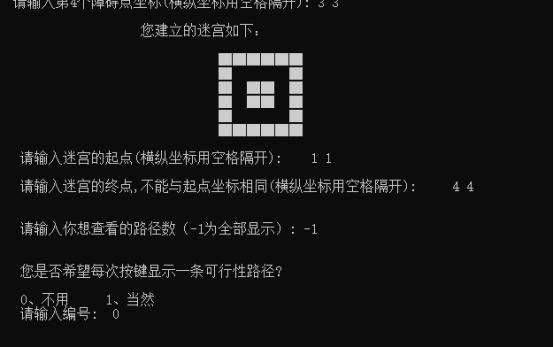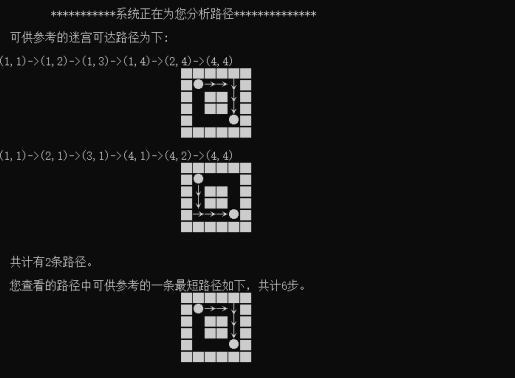异常数据测试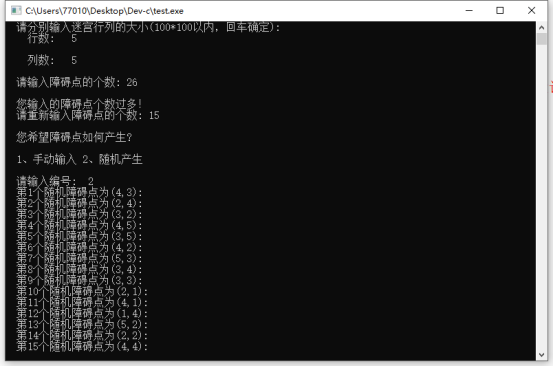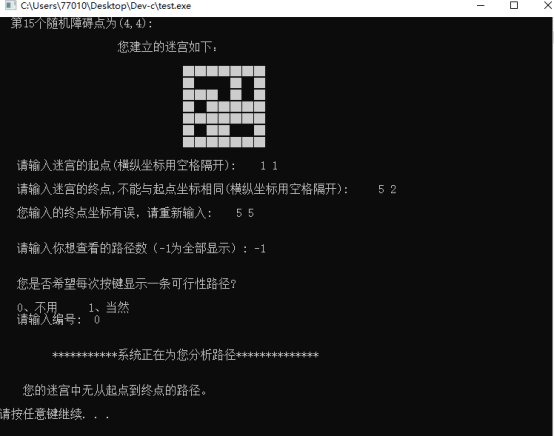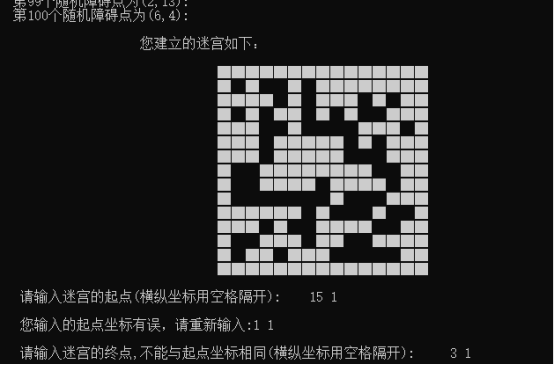有疑问看这里
如果有疑问，请在评论区留言或私信笔者。
源码及课程设计报告点击此处下载：迷宫非递归求解+详细报告
内容含源码一份，课程设计详细报告一份。
提取码：7z7y。
对您有帮助的话，点个赞吧！


展开全文c++ 算法
• 非递归：行列为整型，坐标为整型递归：迷宫以整型二维数组形式输入输出的形式：非递归输出三元组通路和方阵通路； 递归以方阵输出迷宫和所有通路；1、非递归算法，求一条通路输出三元组形式如：（1，1，1）
1.1问题描述
以一个m*n的长方阵表示迷宫，0和1分别表示迷宫中的通路和障碍。设计一个程序，对任意设定的迷宫，求出一条从入口到出口的通路，或得出没有通路的结论。

1.2基本要求
输入的形式和范围：
非递归：行列为整型，坐标为整型

递归：迷宫以整型二维数组形式输入

输出的形式：非递归输出三元组通路和方阵通路；
递归以方阵输出迷宫和所有通路；

1、非递归算法，求一条通路输出三元组形式如：（1，1，1），（1，2，2），（2，2，2），（3，2，3），（3，1，2），…和方阵通路；

2、递归算法，求得迷宫中所有可能的通路，以方阵形式输出迷宫及其通路。

大家先看一个特例：（特例结束后给处通用代码：代码转自AHU15计算机科学与技术专业赵吴攀先生，在此鸣谢）

#include<iostream>
#include<cstdio>
#include<stack>
using namespace std;

typedef struct{
int ord;
int flag;
int i;
int j;
}SElemType;
void di(int &t,int &i,int &j)
{
if(t==1)
{
j=j+1;
}
if(t==2)
{
i=i+1;
}
if(t==3)
{
j=j-1;
}
if(t==4)
{
i=i-1;
}

}
void ri(int &t,int &m,int &n,int i,int j)
{
if(t==1)
{
n=j+1;
}
if(t==2)
{
m=i+1;
}
if(t==3)
{
n=j-1;
}
if(t==4)
{
m=i-1;
}

}
int main()
{
int maze=
{
{1,1,2,3,4,5,6,7,8,9},
{1,0,0,1,0,0,0,1,0,1},
{2,0,0,1,0,0,0,1,0,1},
{3,0,0,0,0,1,1,0,0,1},
{4,0,1,1,1,0,0,0,0,1},
{5,0,0,0,1,0,0,0,0,1},
{6,0,1,0,0,0,1,0,0,1},
{7,0,1,1,1,0,1,1,0,1},
{8,1,0,0,0,0,0,0,0,1},
{9,1,1,1,1,1,1,1,1,1}
};
int curstep=1,m=1,n=1;
int tag=0;
SElemType e;
e.flag=1;e.i=1,e.j=1;
stack<SElemType>S;
do
{
if(maze[m][n]<=0)
{
maze[m][n]=1;
e.ord=curstep;
e.i=m;e.j=n;
e.flag=1;
S.push(e);
if(m==8&&n==8){tag=1;break;}
else{
di(e.flag,m,n);
printf("%d %d\n",m,n);
curstep++;
}
}
else{
if(!S.empty()){
e=S.top();
S.pop();
while(e.flag==4&&!S.empty()){
maze[m][n]=1;
e=S.top();
S.pop();
}
if(e.flag<4){
e.flag++;
S.push(e);
ri(e.flag,m,n,e.i,e.j);
}
}
}
}while(!S.empty());

if(tag==1)
printf("SUCCESS!");
else
printf("ERROR!");

}

递归代码：

#include <stdio.h>
#include <malloc.h>
#define M 6
#define N 6
#define END N-2
int flag=0;
typedef struct
{
int x,y,d;
}position;

/*创建迷宫*/
void creat_maze(int a[][M])
{
int i,j;
for(i=0;i<=N-1;i++)
for(j=0;j<=M-1;j++)
scanf("%1d",&a[i][j]);
}

position nextq(int maze[][M],position q)
{
if(q.d==0)
q.y++;
else if(q.d==1)
q.x++;
else if(q.d==2)
q.y--;
else if(q.d==3)
q.x--;
return q;
}
void initialmat(int route[][M])       //初始化输出路径图
{
int i,j;
for(i=0;i<N;i++)
for(j=0;j<M;j++)
route[i][j]=1;
}
void print_maze(int mat[][M])        //输出迷宫
{
int i,j;
for(i=0;i<N;i++)
{
for(j=0;j<M;j++)
printf("%d ",mat[i][j]);
printf("\n");
}

}
void MazePath(int maze[][M],int route[][M],position ps)
{
position q;
route[ps.x][ps.y]=0;
maze[ps.x][ps.y]=-1;
q=nextq(maze,ps);
q.d=0;
if(q.x==END&&q.y==END)
{
flag++;
printf("\n第%d条路:\n",flag);
route[END][END]=0;
print_maze(route);
route[END][END]=1;
}
else if(maze[q.x][q.y]==0)
MazePath(maze,route,q);
if(ps.d<=3)
{
ps.d++;
MazePath(maze,route,ps);
}
route[ps.x][ps.y]=1;
maze[ps.x][ps.y]=0;
}
void main()
{
int maze[N][M],route[N][M];
position ps;
ps.x=ps.y=1;
ps.d=0;
printf("输入一个迷宫(%d*%d):\n",N,M);
creat_maze(maze);
printf("\n输出该迷宫:\n");
print_maze(maze);
printf("输出从(1,1)到(%d,%d)的所有通路:\n",N-2,M-2);
initialmat(route);
MazePath(maze,route,ps);
}

非递归代码：

#include<stdio.h>
#include<stdlib.h>
#define Elemtype int
#define MAXSIZE 50
typedef struct
{
int x,y;
}mark;               //起点、终点坐标

typedef struct
{
Elemtype x,y;    //迷宫数组坐标(x,y)
int d;    //下一步的方向
}TriMatrix;

typedef struct LStackNode
{
TriMatrix elem;
struct LStackNode *next;
}LStack,*PLStack;

//栈的基本操作

PLStack InitStack(PLStack S)
{
S=NULL;
return S;
}

int StackEmpty(PLStack S)
{
if(S==NULL)  return 1;
else return 0;
}

PLStack Push(PLStack S,TriMatrix e)   //入栈
{
PLStack P;
P=(PLStack)malloc(sizeof(LStack));
P->elem=e;
P->next=S;
S=P;
return S;
}

PLStack Delete(PLStack S)   //删除栈头
{
PLStack P;
P=S;
if(!StackEmpty(S))
{
S=S->next;
free(P);
return S;
}
}

TriMatrix Pop(PLStack S,TriMatrix e)  //出栈
{
if(!StackEmpty(S))
{
e=S->elem;
return e;
}
}

void MazePath(mark start,mark end,
Elemtype maze[MAXSIZE][MAXSIZE],
int m,int n,int diradd)   //寻找路径
{
int i,j,d;
int a,b;
TriMatrix elem,e;
PLStack S1,S2;
S1=InitStack(S1);
S2=InitStack(S2);
maze[start.x][start.y]=2;   //修改入口坐标，做标记
elem.x=start.x;
elem.y=start.y;
elem.d=0;  //开始为0
S1=Push(S1,elem);
while(!StackEmpty(S1))  //栈不空，有路可走
{
elem=Pop(S1,elem);
S1=Delete(S1);
i=elem.x;
j=elem.y;
d=elem.d+1;
while(d<=4)
{
a=i+diradd[d-1];
b=j+diradd[d-1];
if(a==end.x&&b==end.y&&maze[a][b]==0)  //出口
{
elem.x=i;
elem.y=j;
elem.d=d;
S1=Push(S1,elem);
elem.x=a;
elem.y=b;
elem.d=0;   //方向输出为0，判断是否为出口
S1=Push(S1,elem);
printf("\n1=东 2=南 3=西 4=北 0为走出迷宫\n\n路径为:(行坐标，列坐标，方向)\n");
while(S1)  //逆置序列 并输出路径
{
e=Pop(S1,e);
S1=Delete(S1);
S2=Push(S2,e);
}
while(S2)
{
e=Pop(S2,e);
S2=Delete(S2);
printf("-->(%d,%d,%d)",e.x,e.y,e.d);maze[e.x][e.y]=3;
}
printf("\n\n");
for(i=0;i<=m+1;i++){
for(j=0;j<=n+1;j++)
if(maze[i][j]==3) printf("0 ");
else printf("1 ");
printf("\n");
}
return;
}//if
if(maze[a][b]==0)
{
maze[a][b]=2;
elem.x=i;
elem.y=j;
elem.d=d;
S1=Push(S1,elem);
i=a;  j=b;  d=0;
}
d++;
}
}
printf("没有出迷宫的路径\n");
}

//建立迷宫
void initmaze(int maze[MAXSIZE][MAXSIZE],int m,int n)
{
int i,j;
for(i=1;i<=m;i++)
for(j=1;j<=n;j++)
maze[i][j]=rand()%2;
for(i=0;i<=m+1;i++)
{
maze[i]=maze[i][n+1]=1;
}
for(j=0;j<=n+1;j++)
{
maze[j]=maze[m+1][j]=1;
}
printf("输出迷宫:\n");
for(i=0;i<=m+1;i++)
{
for(j=0;j<=n+1;j++)
printf("%d ",maze[i][j]);
printf("\n");
}
}

void main()
{
int maze[MAXSIZE][MAXSIZE];
mark start,end;
int m,n;  //迷宫行列
printf("输入迷宫的行数m和列数n:\n");
scanf("%d%d",&m,&n);
int add={{0,1},{1,0},{0,-1},{-1,0}};
initmaze(maze,m,n);
printf("输入入口坐标和出口坐标:\n");
scanf("%d%d%d%d",&start.x,&start.y,&end.x,&end.y);
MazePath(start,end,maze,m,n,add);
}
展开全文数据结构
•  这个程序为前述迷宫非递归实现，是在将前述栈实现的基础上进行少许更改实现的(此处的更改也即上述程序的一些不足)      问题C语言中传送二位数组的方式. 开始一直出错，最后经测试...
     重新编玩上一程序，想把以前学的迷宫又实现了一遍， 只不过这次编的感受很不同。
这个程序为前述迷宫的非递归实现，是在将前述栈实现的基础上进行少许更改实现的(此处的更改也即上述程序的一些不足)

问题： C语言中传送二位数组的方式.  开始一直出错，最后经测试格式应为 char array[][agru];   //agru为数组的列数

//迷宫的实现方式如下

//主程序
/*
*	文件名: main.cpp
*	功能: 主函数检测实现结果
*	作者: shaofeng5000
*	时间: 2011/4/3
*/

#include <stdio.h>
#include "stack.h"
#include "maze.h"

//迷宫矩阵
char maze;

int main()
{
//创建用来存放路径的栈
PSTACK path;
path = STACK_Create();
if (NULL == path)
{
printf("迷宫初始化失败!");
}
//迷宫大小选定
//初始默认为12 此处固定为12是为了简化测试
int n;
n = 12;

//初始化迷宫，此处迷宫是由文件读入
int i, j;
char ch;
FILE *file = fopen("maze.txt", "r");
if (NULL == file)
{
printf("迷宫初始化失败!");
return 0;
}
for (i = 0; i < n; i++)
{
for (j = 0; j < n; j++)
{
fread(&maze[i][j], 1, 1, file);
}
fread(&ch, 1, 1, file);	//换行符
}

//打印原始迷宫迷宫
for (i = 0; i < n; i++)
{
for (j = 0; j < n; j++)
{
printf("%c ", maze[i][j]);
}
printf("/n");
}

//查找迷宫路径(本测试程序的主题部分)
MAZENODE curNode;	//栈中路径顶的节点
MAZENODE nextNode;	//栈顶节点的试探信息
//让入口位置入栈
curNode.dir = RIGHT;
curNode.i = 1;
curNode.j = 0;
maze = SEEK;	//将迷宫此处改为已找到路径
STACK_Push(path, curNode);	//压入栈中

BOOL bPath = FALSE;		//试探方向是否为可走路径
BOOL bNew = TRUE;	//位置是否为第一次到达
BOOL bFind = FALSE;	//是否找到路径
while (TRUE)
{
if (TRUE == bNew)	//第一次到达位置初始化试探方向
{
BeginStepDir(curNode, &nextNode);
}
else	//转入下一方向
{
NextStepDir(curNode, &nextNode);
}

bPath = CheckPath(maze, nextNode);	//检测此位置是否可走
if (TRUE == bPath)	//可走
{
STACK_Push(path, nextNode);	//将此位置压入路径战中
maze[nextNode.i][nextNode.j] = SEEK;	//将此位置标志为路径
//更该当前节点
curNode.dir = nextNode.dir;
curNode.i = nextNode.i;
curNode.j = nextNode.j;

if (n - 2 == curNode.i && n - 1 == curNode.j)	//到达出口
{
bFind = TRUE;	//标示找到
break;
}
else if (1 == curNode.i && 0 == curNode.j)	//回到入口
{
bFind = FALSE;	//标示未找到
break;
}

bNew = TRUE;	//此位置第一次到达
}
else
{
if (TOP == nextNode.dir)	//所有方向已试探不可
{
maze[curNode.i][curNode.j] = DEAD;	//此位置为死路

STACK_Pop(path, NULL);	//弹出死位置
STACK_GetTop(path, &curNode);	//更改当前位置
//将试探位置改为当前位置 (这样的结果是此位置比较了两次)
nextNode.dir = curNode.dir;
nextNode.i = curNode.i;
nextNode.j = curNode.j;
}

bNew = FALSE;
}
}
printf("n");
for (i = 0; i < n; i++)
{
for (j = 0; j < n; j++)
{
if (maze[i][j] != SEEK)
{
printf("%c ", maze[i][j]);
}
else
{
printf("  ");	//将找到的路径标志为空
}
}
printf("/n");
}

return 0;
}

//迷宫头文件
/*
*	文件名: maze.h
*	功能: 迷宫相关操作
*	作者: shaofeng5000
*	时间: 2011/4/3
*/

#ifndef _MAZE_H
#define _MAZE_H
//默认格式定义
#define BOOL int
#define TRUE 1
#define FALSE 0
//属性
#define	BRICK '='	//砖块
#define PASS  'o'	//通道
#define	DEAD  '+'	//死路
#define SEEK  'p'	//找到的路径
//方向声明
enum DIRECTION
{
RIGHT,	//右方
BOTTOM, //下方
LEFT,	//左方
TOP		//上方
};
//迷宫的数据结构
typedef struct _MAZENODE
{
int i;
int j;
int dir;
}MAZENODE;

//迷宫操作
//开始走
void BeginStepDir(MAZENODE curNode, MAZENODE *nextNode);
//下一方向
void NextStepDir(MAZENODE curNode, MAZENODE *nextNode);
//检测路径
BOOL CheckPath(char maze[], MAZENODE nextNode);

#endif

//迷宫操作实现文件
/*
*	文件名: maze.h
*	功能: 迷宫相关操作
*	作者: shaofeng5000
*	时间: 2011/4/3
*/

#include "maze.h"

/*
功能: 开始试探可走方向
参数: curNode 查找路径最顶端得节点
nextNode 试探方向
返回值: NULL
*/
void BeginStepDir(MAZENODE curNode, MAZENODE *nextNode)
{
//顶端节点向右为第一试探方向
nextNode->dir = RIGHT;
nextNode->i = curNode.i;
nextNode->j = curNode.j + 1;
}

/*
功能: 试探下一方向
参数: curNode 查找路径最顶端得节点
nextNode 试探方向
返回值: NULL
*/
void NextStepDir(MAZENODE curNode, MAZENODE *nextNode)
{
switch (nextNode->dir)
{
case RIGHT:   //方向向下
{
nextNode->dir = BOTTOM;
nextNode->i = curNode.i + 1;
nextNode->j = curNode.j;
}
break;

case BOTTOM:	//方向向左
{
nextNode->dir = LEFT;
nextNode->i = curNode.i;
nextNode->j = curNode.j - 1;
}
break;

case LEFT:     //方向向上
{
nextNode->dir = TOP;
nextNode->i = curNode.i - 1;
nextNode->j = curNode.j;
}
}
}
/*
功能: 检查试探方向是否可走
参数: maze 迷宫
nextNode 试探方向
返回值: 试探方向可走返回TRUE,否则返回FALSE
*/
BOOL CheckPath(char maze[], MAZENODE nextNode)
{
switch (maze[nextNode.i][nextNode.j])
{
case BRICK:	//砖头 不可走
return FALSE;

case PASS:	//通道 可走
return TRUE;

case DEAD:	//死路 不可走
return FALSE;
}
}

//栈头文件
/*
文件名: stack.h
功能: 单链表实现简单的栈
作者: shaofeng5000
时间: 2011/4/3
*/
#ifndef _STACK_H
#define _STACK_H

#include "maze.h"
//默认格式定义
#define BOOL int
#define TRUE 1
#define FALSE 0
#define DATA_TYPE MAZENODE
//栈节点的数据结构
typedef struct _STACKNODE
{
DATA_TYPE data;
struct _STACKNODE *next;
}STACKNODE, *PSTACKNODE;
//栈的数据结构
typedef struct _STACK
{
PSTACKNODE head;	//栈顶指针
int height;	//栈的高度
}STACK, *PSTACK;
//
//栈的基本操作
//
//栈的创建
PSTACK STACK_Create();
//栈的销毁
void STACK_Destroy(PSTACK *stack);
//栈的清空
void STACK_Clear(PSTACK stack);
//得到栈顶
void STACK_GetTop(PSTACK stack, DATA_TYPE *getData);
//压栈操作
BOOL STACK_Push(PSTACK stack, DATA_TYPE pushData);
//弹栈操作
void STACK_Pop(PSTACK stack, DATA_TYPE *popData);

#endif

//栈实现文件
/*
*	文件名: stack.cpp
*	功能: 单链表实现简单的栈
*	作者: shaofeng5000
*	时间: 2011/4/3
*/

#include <stdio.h>
#include <stdlib.h>
#include "stack.h"
/*
功能: 创建栈
参数: NULL
返回值: 指向创建栈的指针
*/
PSTACK STACK_Create()
{
PSTACK stack;
//分配栈结构空间
stack = (PSTACK)malloc(sizeof(STACK));
if (NULL == stack)
{
return NULL;
}
//初始化栈数据
stack->head = NULL;
stack->height = 0;

return stack;
}

/*
功能: 销毁一个栈
参数: stack 指向将要销毁栈的指针
返回值: NULL
*/
void STACK_Destroy(PSTACK *stack)
{
//有效性检验
if (NULL == *stack)
{
return ;
}
//清空栈内节点
STACK_Clear(*stack);
//销毁栈的数据结构
free(*stack);
*stack = NULL;
}

/*
功能: 清空站内节点
参数: stack 指向将要清空栈的指针
返回值: NULL
*/
void STACK_Clear(PSTACK stack)
{
//有效性检验
if (NULL == stack)
{
return ;
}

DATA_TYPE data;
//弹出所有的节点
while (stack->height != 0)
{
STACK_Pop(stack, &data);
}
}

/*
功能: 得到栈顶数据
参数: stack 指向将要操作的栈指针
返回值: NULL
*/
void STACK_GetTop(PSTACK stack, DATA_TYPE *getData)
{
//有效性检查
if (NULL == stack)
{
return ;
}
//得到数据
getData->dir = stack->head->data.dir;
getData->i = stack->head->data.i;
getData->j = stack->head->data.j;
}

/*
功能: 压入栈
参数: stack 指向将要操作的栈的指针
pushData 将要压入的数据
返回值: 压入成功返回TRUE，否则返回FALSE
*/
BOOL STACK_Push(PSTACK stack, DATA_TYPE pushData)
{
//有效性检验
if (NULL == stack)
{
return FALSE;
}

//分配新节点的内存
PSTACKNODE pNewNode;
pNewNode = (PSTACKNODE)malloc(sizeof(STACKNODE));
if (NULL == pNewNode)
{
return FALSE;
}
pNewNode->next = NULL;
//压入栈
pNewNode->data.dir = pushData.dir;
pNewNode->data.i = pushData.i;
pNewNode->data.j = pushData.j;
pNewNode->next = stack->head;
stack->head = pNewNode;

stack->height++;

return TRUE;
}

/*
功能: 弹出栈
参数: stack 将要操作栈的指针
popData 返回弹出栈的数据
返回值:	NULL

*/
void STACK_Pop(PSTACK stack, DATA_TYPE *popData)
{
//有效性检查
if (NULL == stack && stack->height != 0)
{
return ;
}
//弹栈操作
PSTACKNODE pNode;
pNode = stack->head;
stack->head = stack->head->next;

stack->height--;
//内存释放
free(pNode);
pNode = NULL;
}


展开全文null 数据结构 path struct file
• 虽然递归实现起来简单易理解，但使用递归并不是唯一的选择，而且，使用递归总给人一种效率可能不高的感觉。通过简单改造，我们也可以使用非递归的方法来实现回溯法。具体思路是： 为了保存每一步的“进度”，可以...
• 首先实现一个以链表作存储结构的栈类型，然后编写一个求解迷宫问题非递归算法。求得的通路以三元组（i,j,d）的形式输出，其中：(i,j)指示迷宫中的位置，d表示走到下一个坐标的方向。如：对于下列数据的迷宫，输出...
• 非递归方法实现迷宫问题C语言，对最短路径进行标注，方法基础，适合入门者
• 基本要求：首先实现一个以链表作存储结构的栈类型，然后编写一个求解迷宫非递归程序。求得的通路以三元组（i,j,d）的形式输出，其中：（i,j）指示迷宫中的一个坐标，d表示走到下一坐标的方向。如：对于下列数据的...
• 迷宫问题.txt 逆波兰计算器.txt 逆矩阵.txt 逆阵.txt 递堆法.txt 递归桃猴.txt 递归车厢.txt 递推.txt 逻辑移动.txt 链串.txt 链栈.txt 链表十五人排序.txt 链表（递归）.txt 链队列.txt 队列.txt ...数据结构
• 迷宫问题.txt 逆波兰计算器.txt 逆矩阵.txt 逆阵.txt 递堆法.txt 递归桃猴.txt 递归车厢.txt 递推.txt 逻辑移动.txt 链串.txt 链栈.txt 链表十五人排序.txt 链表（递归）.txt 链队列.txt 队列.txt ...
• 迷宫问题.c 逆波兰计算器.c 递归车厢.c 队列.c ./数据结构/单链表: ww.c 冒泡排序.c 单链表1.c 单链表2.c 单链表.c 单链表倒序.c 单链表的处理全集.c 建立链表1.c 节点.c 质因子.c 链表十五人排序.c 链表（递归...
• 112 求解线性方程 113 实矩阵乘法运算 114 求解线性方程 115 n阶方阵求逆 116 复矩阵乘法 117 求定积分 118 求满足特异条件的数列 119 超长正整数的加法 第四部分 图形篇 120 绘制直线 121 绘制圆 ...
• 走迷宫(C语言)迷宫问题【问题描述】 以一个m*n的长方阵表示迷宫，0和1分别表示迷宫中的通路和障碍。设计一个程序，对任意设定的迷宫，求出一条从入口到出口的通路，或得出没有通路的结论。 【基本要求】首先实现一个...
• 112 求解线性方程 113 实矩阵乘法运算 114 求解线性方程 115 n阶方阵求逆 116 复矩阵乘法 117 求定积分 118 求满足特异条件的数列 119 超长正整数的加法 第四部分 图形篇 120 绘制直线 121 绘制圆 ...
• c语言开发实例目录： 第一部分 基础篇 001 第一个C程序 002 运行多个源文件 003 求整数之积 004 比较实数大小 005 字符的输出 006 显示变量所占字节数 007 自增/自减运算 008 数列求和 009 乘法口诀表 010 猜数字...
• C语言实例解析精粹》(曹衍龙 & 林瑞仲 & 徐慧)清晰版[PDF] 状态: 精华资源 图书分类: 教育/科技 出版社: 人民邮电出版社 发行时间: 2007年8月1日 语言: 简体中文 时间: 6月18日 发布 | 6月18日 更新 分类: ...
• 03-002栈的应用、数制转换、括号匹配、行编辑问题、迷宫问题 03-003栈的应用：表达式求值、后缀表达式的表示 03-004队列的定义与存储、顺序队列、链式队列、循环队列 04-001串的定义、表示与实现 04-002串的模式...
• 112 求解线性方程 113 实矩阵乘法运算 114 求解线性方程 115 n阶方阵求逆 116 复矩阵乘法 117 求定积分 118 求满足特异条件的数列 119 超长正整数的加法 第四部分 图形篇 120 绘制直线 121 绘制圆 ...
• 112 求解线性方程 113 实矩阵乘法运算 114 求解线性方程 115 n阶方阵求逆 116 复矩阵乘法 117 求定积分 118 求满足特异条件的数列 119 超长正整数的加法 第四部分 图形篇 120 绘制直线 121 绘制圆 ...
• 112 求解线性方程 113 实矩阵乘法运算 114 求解线性方程 115 n阶方阵求逆 116 复矩阵乘法 117 求定积分 118 求满足特异条件的数列 119 超长正整数的加法 第四部分 图形篇 120 绘制直线 121 绘制圆 ...
• 112 求解线性方程 113 实矩阵乘法运算 114 求解线性方程 115 n阶方阵求逆 116 复矩阵乘法 117 求定积分 118 求满足特异条件的数列 119 超长正整数的加法 第四部分 图形篇 120 绘制直线 ...
• 112 求解线性方程 113 实矩阵乘法运算 114 求解线性方程 115 n阶方阵求逆 116 复矩阵乘法 117 求定积分 118 求满足特异条件的数列 119 超长正整数的加法 第四部分 图形篇 120 绘制直线 121 绘制圆 ...源代码
• C语言实例解析精粹(第二版) 光盘代码 本文件包括以下内容： ※ 1、文件说明 ※ 2、源码操作说明 ※ 3、光盘目录清单 ◎ 源码操作说明 源代码使用方法是（以实例1为例）： 将该实例的源码，比如实例1的1.c文件（可以...# c语言非递归实现迷宫问题c语言 订阅